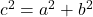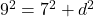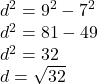Logan is a land surveyor. He wants to determine d, the distance across a lake that cannot be directly measured. He draws this diagram to hel

Question

Logan is a land surveyor. He wants to determine d, the distance across a lake that cannot be directly measured. He draws this diagram to help him.

A right triangle with side length 7 kilometers, d, and hypotenuse of 9 kilometers.

What is the approximate distance across the lake, to the nearest tenth of a kilometer?
5.7 km
6.3 km
11.4 km
16.0 km

in progress 0
6 months 2021-07-21T15:38:52+00:00 2 Answers 29 views 0

The first option     (5.7km)

Step-by-step explanation:

Since we have a right angle triangle and we know two of its sides we can easily find out the thirds side (the value of d) by using the Pathagoras Theorem. In our case 7 and d are our legs and the hypotenuse is equal to 9, so…

(Based on the Pathagoras Theorem)d ≈ 5.7km

There for aproximate distance across the lake is equal to 5.7km